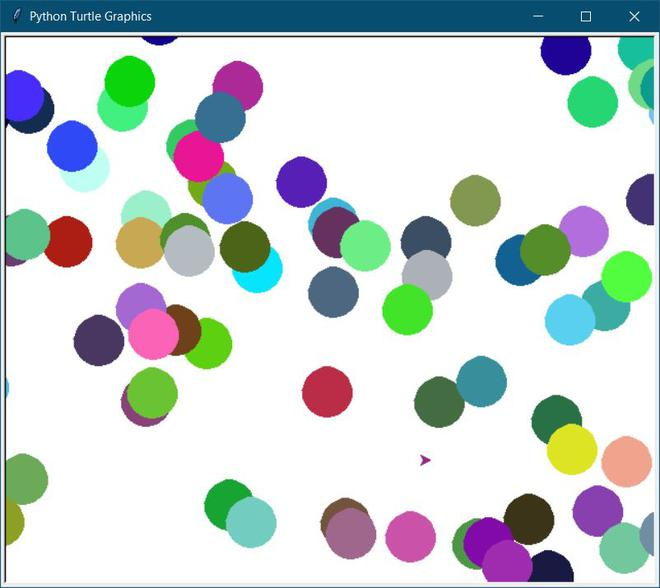Open in App
Not now

# How to make random colors in Python – Turtle?

• Last Updated : 30 Aug, 2022

The turtle is a built-in module from the Python library. The turtle module is used to draw interesting shapes or drawings. We can use the turtle module by calling import turtle. The random module is used to generate random numbers.

### Methods Used

• randint(0,255): It is used to generate numbers between 0 and 255.
• speed(0): It is used to set speed to display the drawing on board.
• colormode(255): It should be set to 255 to generate a color number till 255.
• begin_fill(): It begins to fill the circle with color.
• end_fill(): It ends to fill the circle with color.
• penup(): It will stop drawing on the board.
• pendown(): Turtle operates with pendown() state by default. To go back to the past drawing state on board.
• circle(radius): It is used to generate a circle of a particular radius.

All the above methods will be called inside an infinite loop in order to illustrate a randomly generated coloured circles of the same radius.

Below is the implementation.

## Python3

 `# import turtle``from` `turtle ``import` `*``# import random``from` `random ``import` `randint`  `# speed to draw to color``speed(``0``)` `# size of the pen``pensize(``10``)` `# colormode should be 255 to``# show every type of color``colormode(``255``)`  `# To display the color continuously the``# while loop is true``while` `True``:``    ` `    ``# randint will have random color based on``    ``# every randint the color will be called``    ``color(randint(``0``, ``255``),``          ``randint(``0``, ``255``),``          ``randint(``0``, ``255``))``    ` `    ``# it will begin to fill the circle with color``    ``begin_fill()``    ` `    ``# generate circle``    ``circle(``20``)``    ` `    ``# it will end to fill color``    ``end_fill()``    ` `    ``# it will start to draw``    ``penup()``    ` `    ``# x axis and y axis``    ``goto(randint(``-``500``, ``500``), randint(``-``300``, ``270``))``    ` `    ``# it will stop to draw``    ``pendown()`

OutputRandom color

My Personal Notes arrow_drop_up